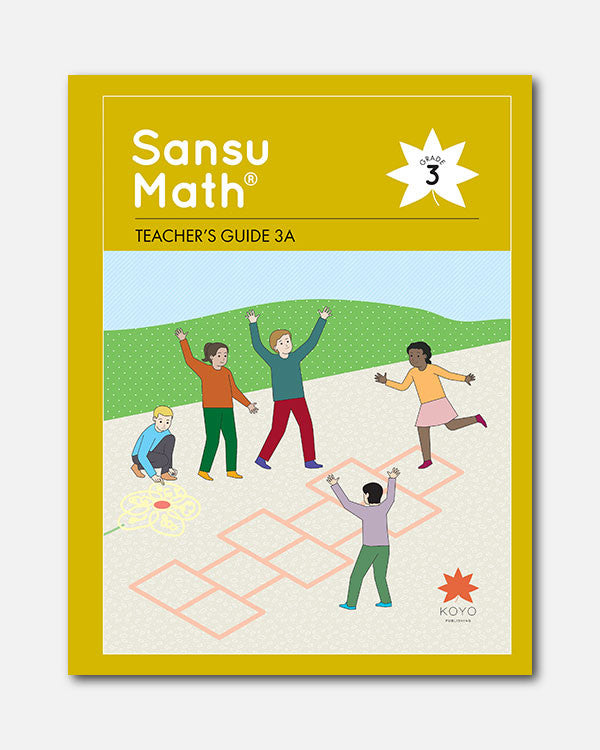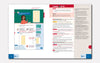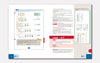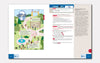# Sansu Math® Teacher's Guide 3A

In Stock
\$ 35.00

### Overview

Each unit of the Teacher's Guide begins with a unit overview that provides a discussion of core mathematical ideas, explanation of the learning trajectory for the unit and for the topic (across units), identification of key points, and ways to support students having difficulties with the unit.

The Teacher's Guides include full-color images of each page of the student textbook with overlaid answers, mirrored by a teacher’s page with lesson notes. These provide specific pedagogical suggestions, including hatsumon (key questions or problems to pose to students), anticipated responses, strategies to support student thinking, and assessment perspectives.

Lesson plans are complemented by carefully-crafted instructional boards which guide students through the units. The Teacher's Guides place an emphasis on recognizing the student's experience, incorporating varied perspectives from student characters and encouraging young learners to make discoveries on their own.

Teacher's Guides are spiral bound books designed to lay flat, and include examples of blackboard organization that can be projected using classroom technology.

Sansu Math® Teacher's Guide 3A is written for use with Sansu Math® Grade 3 student textbook for units 1-10.

1. Multiplication
1. Properties of Multiplication
2. Multiplication with 0
2. How to Find Time and Elapsed Time
1. How to Find Time and Elapsed Time
2. Shorter Periods of Time
3. Division
1. Calculations for Finding How Many for 1 Person
2. Calculations for Finding the Number of People We Can Divide Something Into
3. Calculations for Finding Times as Much
4. Circles and Spheres
1. Circles
2. Spheres
5. Addition and Subtraction Algorithms
1. Addition of 3-Digit Numbers
2. Subtraction of 3-Digit Numbers
3. Calculations of Large Numbers with the Algorithm
6. Mental Calculation
7. Division with Remainders
1. Division with Remainders
2. Problems Dealing with Remainders
8. Structure of Large Numbers
1. How to Express Numbers
2. Numbers Multiplied by 10 and Divided by 10
9. Multiplication Algorithm (1)
1. Multiplication by 10 and 100
2. Multiplication of 2-Digit Numbers by 1-Digit Numbers
3. Multiplication of 3-Digit Numbers by 1-Digit Numbers
4. Calculations with Times as Much
10. Division With Large Numbers
Additional Problems for Units 1-10